Algebra

Equations

Inequalities

Graphs

Numbers

Calculus

Matrices

Tutorials

# Deﬁnitions, properties and solution of linear inequalities in one variable

Deﬁnitions and Notation

When the real numbers a and b are represented by points on a number line, one of the following relationships hold:

1. When the graph of a lies to the right of the graph of b, then a is greater than b, denoted by a>b.

2. When a and b represent the same point, then a equals b, denoted by a=b.

3. When the graph of a lies to the left of the graph of b, then a is less than b, denote by a<b.

Note that the statements a>b and b<a are equivalent.

Examples   1.  5 lies to the right of 2,5>2.

2.   8 lies to the left of 6, 8< 6.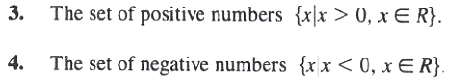The statement 10>4 means that if we subtract 4 from 10, we get a positive number, 10-4 = + 6

The statement 3> 8 means that if we subtract 8 from 3, we get a positive number, ( 3)-( 8) = 3+8 = + 5

Definition   For a,bRa>b means that a-bis a positive number.

If a-b is a positive number, we can write

a-b=k     where a,b,kRk>0

Since a-b=k  and a=b+k are equivalent statements,

a>b  means  a=b+k,  where  k>0

If ais grater than or equal to b, the notation ab is used. Thus ab means either a>bor a=b.

If ais less than or equal to b, the notation ab is used. Thus ab means either ab or a=b

Definition  The relation >,<,≥,≤ are called order relations.

To represent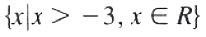graphically. draw a ray from the point whose coordinate is - 3 in the positive direction. Place a hollow circle at the point to denote that the point is not included on the ray (Figure 5.1).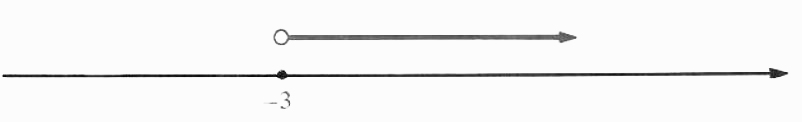Figure 5.1

To represent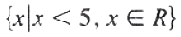graphically, draw a ray from the point whose coordinate is 5 in the negative direction. Place a hollow circle at the point to denote than the point is not included on the ray (Figure 5.2).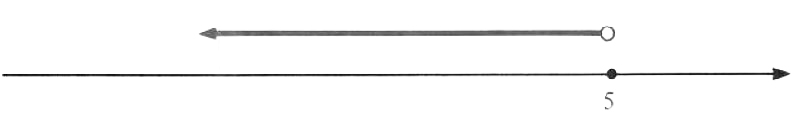Figure 5.2

To represent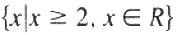graphically. draw a ray from the point whose coordinate is 2 in the positive direction. Place a solid dot at the point to denote that the point is included on the ray (Figure 5,3).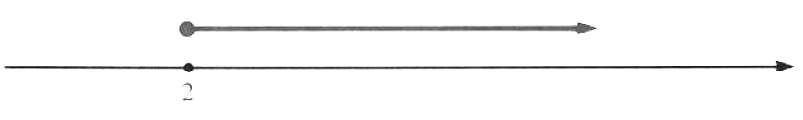Figure 5.3

To represent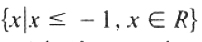graphically, draw a ray from the point whose coordinate is  1 in the negative direction. Place u solid dot at the point to denote that the point is included on the ray (Figure 5.4),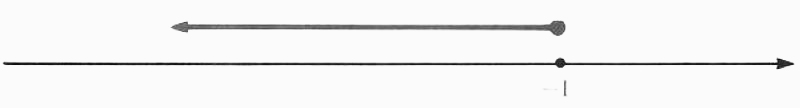Figure 5.4

Properties of the Order Relations

Since a>b and b<a are equivalent statements. from the theorems below for the “greater than” relation we can derive similar theorems for the “less than" relation.

THEOREM 1

Let a,b,cR; if a>b, then a>c.

EXAMPLES  1.    2> 10 and 10> 20;

hence -2 > -20

2.    1<7 and 7<13;

hence  1<13

THEOREM 2

Let a,b,c,dR if a>b and c>d, then a+c>b+d

EXAMPLES  1.   5>2        and 8> 20

5+( 8)= 3   and 2+( 20)= 18

Since 3> 18, then 5+( 8)>2+( 20)

2.    2<0         and 10<14

2+10 = 8   and 0+14 = 14

Since 8<14, then 2+10<0+4

THEOREM 3

Let a,b,cR; if a>b, then a+c>b+c

EXAMPLES  1.   20>7

20+( 30) = 10 and 7+( 30) = 23

Since 10> 23, then 20+( 30)>7+( 30).

2.    10< 2

10+5= 5 and 2+5=3

Since 5<3, then 10+5< 2+5.

THEOREM 4

Let a,b,c,dR, and a,b,c,d>0;

if a>band a>d, then ac>bd

EXAMPLES  1.   7>3 and 8>2

7 (8)=56 and 3 (2)=6

Since 56>6, then 7 (8)>3 (2).

2.   4<9 and 5<7

4 (5)=20 and 9 (7)=63

Since 20<63, then 4 (5)<9 (7).

THEOREM 5

Let a,b,cRc>0; if a>b, then ac>bc

EXAMPLES  1.   5> 2 and 3>0

5 (3)=15 and 2 (3)= 6

Since 15> 6, then 5 (3)> 2 (3).

2.    8< 3 and 5>0

8 (5)= 40 and 3 (5)= 15

Since 40< 15, then 8 (5)< 3 (5).

THEOREM 6

Let a,b,cR,c>0; if a>b, then ac>bc.

EXAMPLES  1.   15>12 and 2<0

15 ( 2)= 30 and 12 ( 2)= 24

Since 30< 40, then 15 ( 2)<12 ( 2).

2.    10<3 and 4<0

10 ( 4)=40 and 3 ( 4)= 12

Since 40> 12, then 10 ( 4)>3 ( 4)

Note

if a,bR and a>b, then  a< b

20>6 hence 20< 6

15> 4 hence 15<4

Solution of Linear Inequalities in One Variable

The following are examples of statements of order of two algebraic expressions

1.  5 x<5 x+2

2.  x2+y20

3.  7 x+2<3 x+18

4.  2 x+9x+20

5.  3 x+73 (x+1)

6.  6 (x+y)>6 x+6 y+1

Statements 1 and 2 are true for all real values of the variables involved. Such statements are called absolute statements.
Statements 3 and 4 are true for some but not all real values of the variable involved. Statement 3 is true when x is less than 5. Statement 4 is true when x is greater than or equal to 11. Such statements are called conditional inequalities or. simply.
inequalities.
Statements 5 and 6 are not true for any real number.

The set of all numbers that satisfy the inequality is called the solution set of the inequality.
Note   A linear equation in one variable has one element in its solution set. The solution set the equation 3 x-4 = 5 is {3}.

A linear inequality in one variable has more than one element in its solution set. The solution set of the inequality 3 x-4>5 is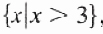, that is, all the real numbers that are greater than 3.

DEFINITION  Two inequalities arc said to be equivalent if they have the same solution set.

To ﬁnd the solution set of an inequality, we must ﬁrst state some theorems.

THEOREM 1  If P.Q. and Tare polynomials in the same. variable and P>Q is an inequality then P>Q and P+T>Q+T are equivalent.

Theorem 1 shows that we can add a polynomial to both sides of the inequality and obtain an equivalent inequality.

EXAMPLE:  1.   If x+7>15

then x+7+( 7)>15+( 7)

x>8

x>8 is equivalent to x+7>15

To ﬁnd the solution set of a linear inequality in one variable, apply the previous theorem to obtain an equivalent inequality of the form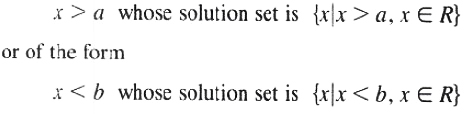Note  When both sides of the inequality contain terms that have the variable as at factor and terms that do not have the variable as a factor. form an equivalent inequality that has at the terms with the variable as a factor on one side and the terms not having the variable on the other side. This can be accomplished by adding the additive inverses (negatives) of the terms to both sides of the inequality.

EXAMPLES  Find the solution set of the inequality

x+5>2

Solution

Adding 5 to both sides of the inequality, we get:

x-5-5>2-5

x> 3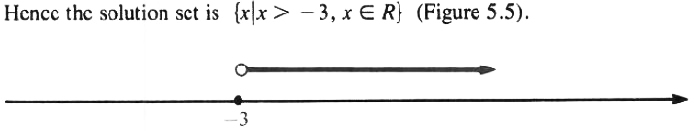FIGURE 5.5

EXAMPLES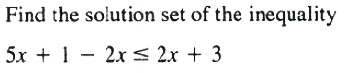Solution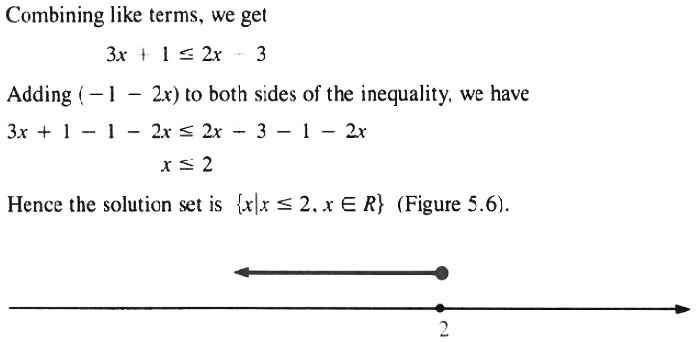FIGURE 5.6

Note  When the inequality involves symbols of grouping. ﬁrst perform the operations that these symbols designate.

EXAMPLES  Find the solution set of the inequality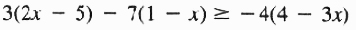Solution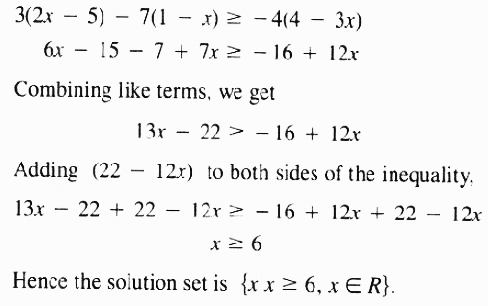EXAMPLES  Find the solution set of the inequality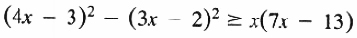Solution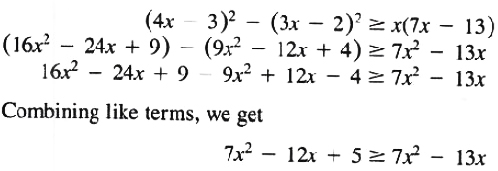to both sides of inequality,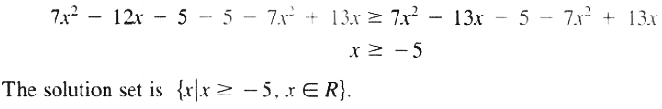EXAMPLES  List the elements in the set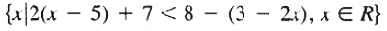Solution    Consider the statement

2 (x-5)+7<8-(3-2 x)

2 x-10+7<8-3+2 x

2 x-2 x<8-3+10-7

0 x<8

Since 0 x<8

is true for all real values of x, we have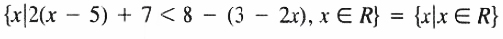EXAMPLES  List the elements in the set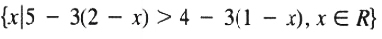Solution    Consider the statement

5-3 (2-x)>4-3 (1-x)

5-6+3 x>4-3+3 x

3 x-3 x>4-3-5+6

0 x>2

Since 0 x>2 in not true for any real value of x we have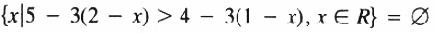THEOREM 2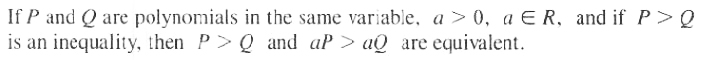Theorem 2 shows that if we multiply both sides of an inequality by a positive real number, we obtain an equivalent inequality.

EXAMPLES  1. 2 x>6

12 (2 x)>12 (6), that is, x>3

x>3 is equivalent to 2 x>6

2. 34 (x)< 9

43 (34 X)<43 ( 9), that is, x< 12

x< 12 and 34 x< 9 are equivalent.

EXAMPLE   Find the solution set of the inequality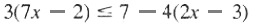Solution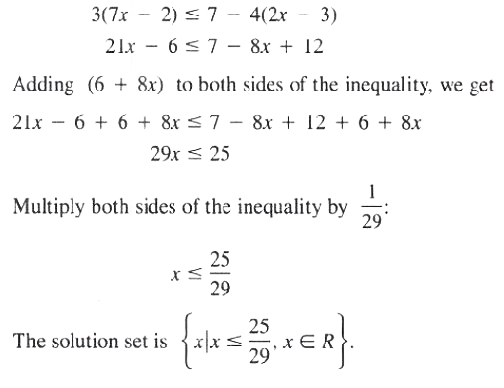EXAMPLE   Find the solution set of the inequality

32 x+16>23 x-23

Solution     Multiply both sides of the inequality by the least common denominator, which is

9 x+1>4 x-4

Adding ( 1-4 x) to both sides, we get

5 x> 5

Multiply both sides of the inequality by 15

x> 1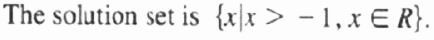THEOREM 3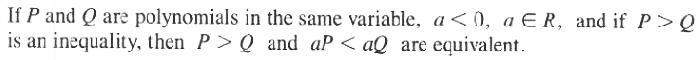Theorem 3 shows that if we multiply both sides of an inequality by a negative number, an equivalent inequality results with the direction of the order relation reversed.

EXAMPLES  1.    5 x>10

(15) ( 5 x)< (15) (10) that is x< 2

x< 2 and 5 x< 10 are equivalent

2.    4 x< 5

(14) ( 4 x)> (14) ( 8), that is x>2

x>2 and 4 x< 8 are equivalent

EXAMPLE    Find the solution set of the inequalitySolution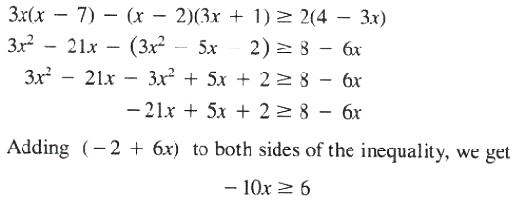Multiply both sides of the inequality by 110 and reverse the direction of the order relation: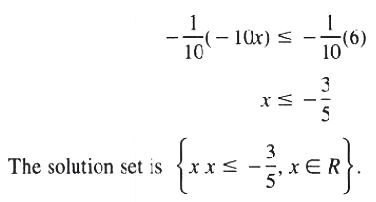EXAMPLE    Find the solution set of the inequality

(4 x+5) (3 x-2)-4 (x+1) (3 x-1)< 6

Solution    (4 x+5) (3 x-2)-4 (x+1) (3 x-1)< 6

12 x2+7 x-10-12 x2-8 x+4< 6

x-6< 6

Add (+ 6) both sides;  x<0

Multiply both sides of the inequality by ( 1) and reverse direction of the order relation:

x>0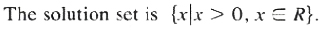← Previous Tutorial Next Tutorial →